# The Vacuum Airship

Archimedes’ Principle states that the (upthrust) force on an object that is displacing a fluid is equal to the weight of the fluid displaced. For example, a cube with sides of one metre, fully submerged in water, will experience an upward force of 9810 newtons, as this is the weight of one cubic metre of water. The material that the object is made of has no effect on this force, so if the object weighs more than 9810 newtons it will sink, and if it weighs less than 9810 newtons it will float.

The upthrust force on an object therefore depends only on the relative densities of the object and the fluid it is displacing. A bigger difference means a bigger force.

Hydrogen is the least dense gas, at 0.0898 kilograms per metre cubed, but hydrogen is rarely used in airships as it is highly flammable and therefore dangerous. Hydrogen was used in the Hindenburg because  helium was difficult to produce and the United States, the only country with significant reserves, had banned its export. Helium has a density of 0.179 kg/m3 and therefore produces only 93% of the lift of hydrogen, but it is far, far safer.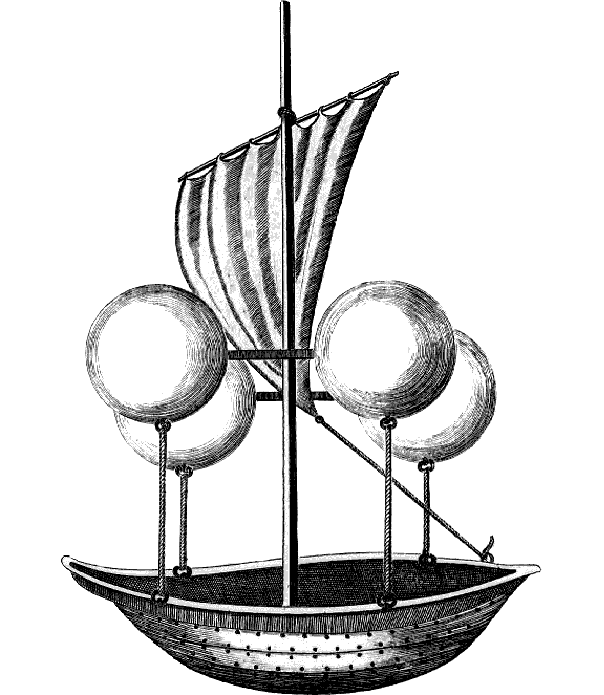Ideally, to create the maximum upthrust force, we would want our balloon or airship’s envelope to be filled with something with the lowest possible density. The lowest possible density would be a vacuum, the total absence of anything, which would create a lifting force of 12.7 newtons per cubic metre (as opposed to 11.0 N/m3 for helium).

The problem with using a vacuum to lift an airship, is how to contain the vacuum. If a difference in pressure exists between two regions, then a difference in force exists between those two regions. In the case of a vacuum airship being used on Earth’s surface, that force would be 101325 newtons per square metre, the equivalent of more than ten tonnes pushing down on every square metre. No material on Earth is strong enough to withstand this force without being so heavy as to negate the point of the vacuum lifting effect in the first place. In order to still have lifting capability and withstand the stresses involved, we can calculate the minimum required ratio of Young’s modulus to density, and this yields a figure of around 450000 Pa/(kg/m3)2. Unfortunately, even the strongest materials, like diamond, have ratios that are only one-fifth of this, so it looks like we won’t be creating vacuum airships any time soon.

# The Kármán Line

The Kármán Line, at 100 kilometres above sea level, is commonly taken to be the boundary between Earth’s atmosphere and space. But why?

An aircraft generates lift by moving through air.$L=\frac{1}{2} AC_L \rho v^2$

where$L$ is the lift generated,$A$ is the wing area,$C_L$ is the lift coefficient,$\rho$ is the density of air, and$v$ is speed through the air.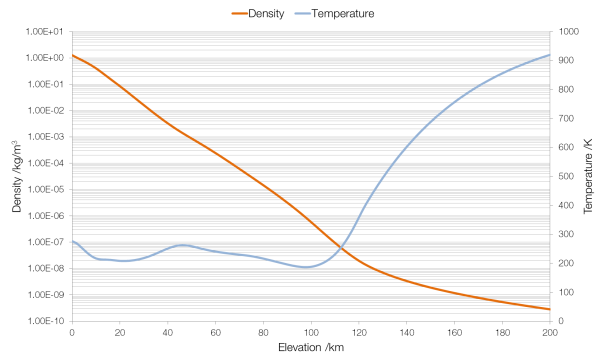Data from NASA MSIS E-90 atmosphere model. Note that density is plotted on a logarithmic scale and (absolute) temperature on a linear scale.

The density of air changes with elevation (altitude is height above ground, elevation is height above sea level). Therefore, keeping all other factors the same (which would be the case for an aircraft, which cannot change the area of its wings or its lift coefficient), as the air becomes less dense an aircraft must increase its speed to stay airborne. A Boeing 747-400 has a wing area (planform) of 541.2 square metres and a lift coefficient of around 0.5. Assuming that it flies empty, with a mass of 178?800 kilograms (weight 1?754?000 N), the speed it will need to fly to generate the required lift changes rapidly as the air becomes thinner. (At an elevation of 200?km a 747-400 would have to fly at nearly twenty thousand times the speed of sound.)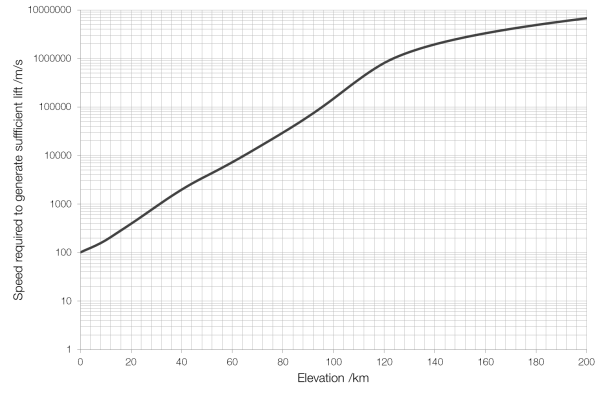Speed required at elevation for a Boeing 747-400.

The cruising speed of a 747-400 is about Mach 0.85 or 290 metres per second, which limits the 747-400 to an elevation of around 16 km. (In reality it flies much lower, around 12?km, for safety and fuel consumption reasons.) The record elevation for sustained horizontal flight by a ground-launched aircraft is 36?240 m by a MiG-25M, which was, at the time, one of the fastest military aircraft with a top speed of Mach 2.83.

The Kármán Line, at an elevation of 100?km, is the point at which the atmosphere is so thin (more than a million times thinner than air at sea level) that an aircraft would have to be moving at a speed faster than it could orbit in order to stay in the air. Thus, it is the greatest altitude at which an aircraft could fly in a straight line rather than following the curvature of the Earth.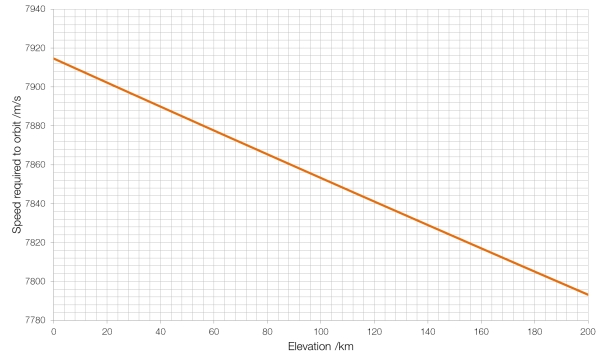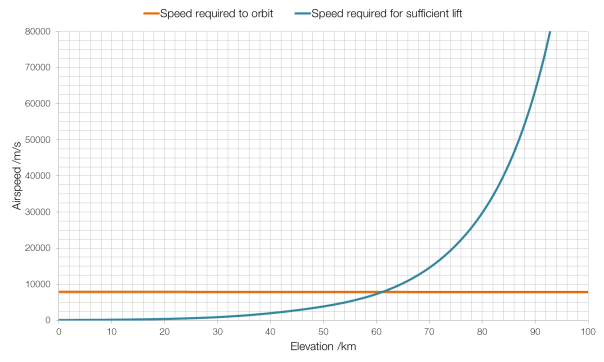As you can see from the graph above, for our Boeing 747-400, the Kármán Line is around 61?km, rather than the full one hundred kilometres, because the 747-400 is not the most aerodynamic of aircraft, designed for bulk passenger carrying and cost-efficiency rather than performance.